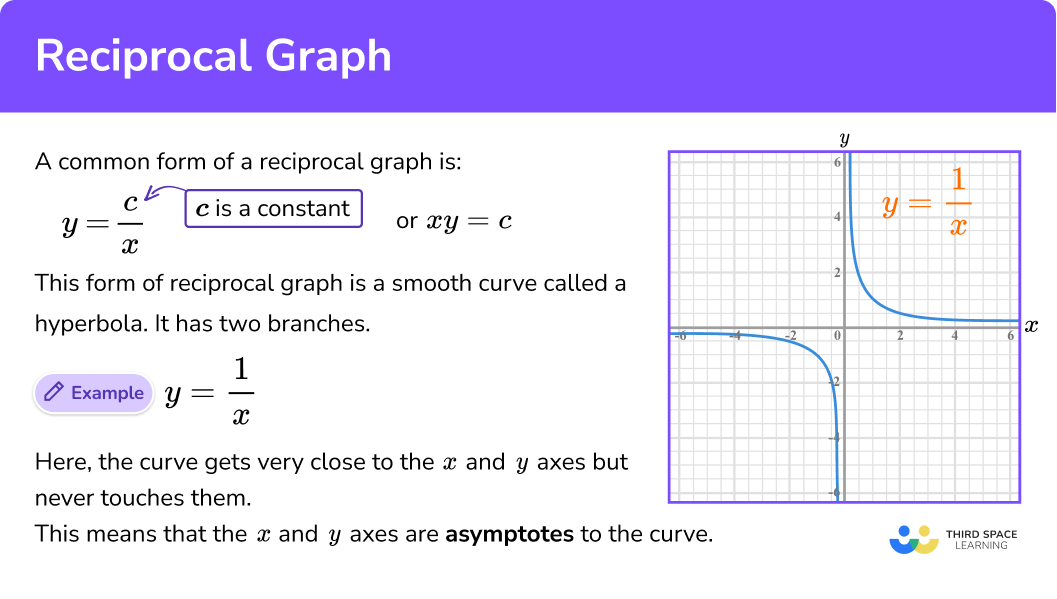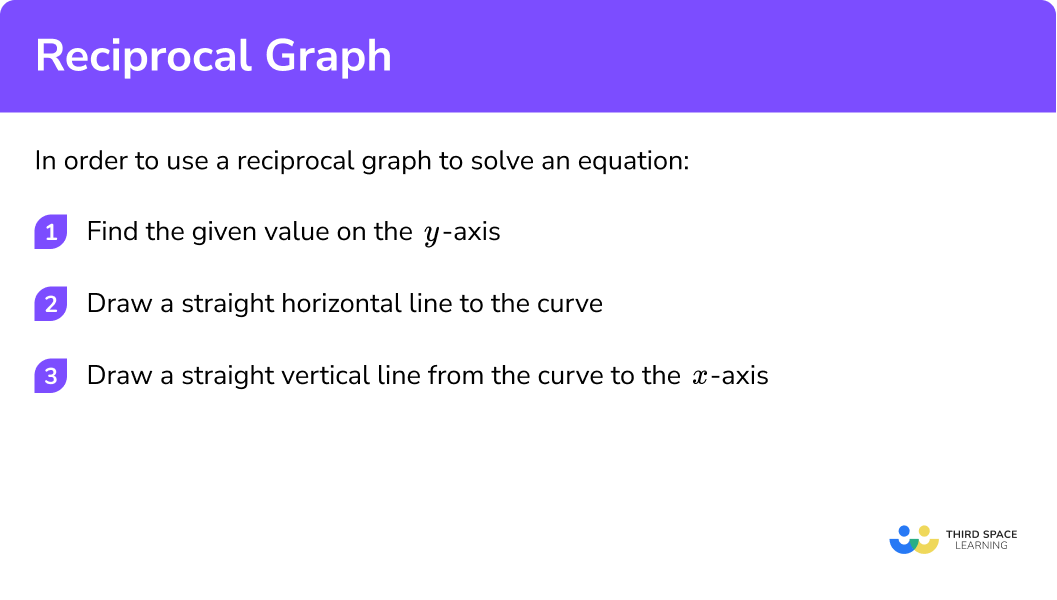# Reciprocal Graph

Here we will learn about reciprocal graphs, including how to recognise and sketch them. We will also look at plotting and interpreting graphs of reciprocal functions.

There are also reciprocal graphs worksheets based on Edexcel, AQA and OCR exam questions, along with further guidance on where to go next if you’re still stuck.

## What is a reciprocal graph?

A reciprocal graph is of the form y = ax, where a is a constant.

E.g. Here is the graph of y = 1x.

The graph is a smooth curve called a hyperbola

We can see that there is a break in the graph when x = 0 . The curve gets very close to the x and y axes but never touches them.

This means that the x and y axes in this example are asymptotes to the curve.

### What is a reciprocal graph?## How to recognise a reciprocal graph

In order to recognise a reciprocal graph:

1. Identify linear or quadratic or any other functions.
2. Identify the reciprocal function.

Reciprocal graph is part of our series of lessons to support revision on types of graphs. You may find it helpful to start with the main types of graphs lesson for a summary of what to expect, or use the step by step guides below for further detail on individual topics. Other lessons in this series include:

## Recognising a reciprocal graph examples

### Example 1: recognise a reciprocal graph

Which is the correct equation for the graph?

1. Identify linear or quadratic or any other functions.

y=x^2+3 is a quadratic function; its graph would be a parabola.

y=3x+1 is a linear function; its graph would be a straight line.

y=3^x is an exponential function; its graph would be a growth curve.

2Identify the reciprocal function.

y=\frac{3}{x} is a function which has x as the denominator so its graph would be a hyperbola.

The correct equation for the graph is:

y=\frac{3}{x}

### Example 2: recognise a reciprocal graph

Which is the correct graph for the equation?

y=\frac{5}{x}

Identify linear or quadratic or any other functions.

Identify the reciprocal function.

## How to use a reciprocal graph

In order to use a reciprocal graph to solve an equation:

1. Find the given value on the y -axis.
2. Draw a straight horizontal line to the curve.
3. Draw a straight vertical line from the curve to the x -axis.
4. Read off the value on the x -axis.

### Explain how to use a reciprocal graph## Using a reciprocal graph examples

### Example 3: using a reciprocal graph

Use the graph of y=\frac{8}{x} to find an approximate solution of the equation.

\frac{8}{x}=6

Find the given value on the y-axis.

Draw a straight horizontal line to the curve.

Draw a straight vertical line from the curve to the x-axis.

Read off the value on the x-axis.

### Example 4: using a reciprocal graph

Use the graph of y=\frac{7}{x-1}+2 to find an approximate solution of the equation.

\frac{7}{x-1}+2=5

Find the given value on the y-axis.

Draw a straight horizontal line to the curve.

Draw a straight vertical line from the curve to the x-axis.

Read off the value on the x-axis.

## How to plot a reciprocal graph

In order to plot a reciprocal graph:

1. Complete the table of values.
2. Plot the coordinates.
3. Draw a smooth curve through the points.

## Plotting a reciprocal graph examples

### Example 5: plot a reciprocal graph

Draw the curve for 1\leq{x}\leq12

y=\frac{12}{x}

Complete the table of values.

Plot the coordinates.

Draw a smooth curve through the points.

### Example 6: plot a reciprocal graph

Draw the curve for 0\leq{x}\leq9

y=\frac{10}{x+1}

Complete the table of values.

Plot the coordinates.

Draw a smooth curve through the points.

### Common misconceptions

• Joining up curves

Curves should be smooth.
Do NOT join up the plotted points with straight lines like this:

The points should be joined by a single, smooth curve.

• Points on the curve

All points should be on the curve. If there is a point that does not join up with the other points when you draw a smooth curve – go back and check the coordinate.
In the example below there is a point that does not lie on the smooth curve so this needs checking.

### Practice reciprocal graph questions

1. Identify the correct equation for the graph:y=x^2+9y=9x+2y=\frac{9}{x}y=9^xThe graph is a hyperbola. We are looking for a function which has x as the denominator.

y=\frac{9}{x} has x as the denominator so it is a reciprocal function and its graph will be a hyperbola.

y=x^2+9 is a quadratic function; its graph will be a parabola.

y=9x+2 is a linear function; its graph will be a straight line.

y=9^x is an exponential function; its graph will be a growth curve.

2. Identify the correct graph for the equation:

y=\frac{5}{x}+1The function which has x as the denominator.  We need the graph of a reciprocal function. We are looking for a hyperbola.

Graph B is the hyperbola.

Graph A is a growth curve; its function will be an exponential function.

Graph C is a straight line; its function will be a linear function.

Graph D is a parabola; its function will be a quadratic function.

3. Identify the correct graph for the equation:

y=\frac{10}{x}A correct table of values would be:

These values give coordinates such as (1,10), (2,5) and so on.

The coordinates are plotted on the grid.

A smooth curve should be drawn through the points.

4. Identify the correct graph for the equation:

y=\frac{8}{x-1}-2A correct table of values would be:

These values give coordinates such as (2,6), (3,2) and so on.

The coordinates are plotted on the grid.

A smooth curve should be drawn through the points.

5. Use the graph of y=\frac{11}{x} to solve the following equation:

\frac{11}{x}=33.7334.15.2Find 3 on the y-axis .  Draw a straight line across to the curve.

Draw a straight line down to the x-axis and read off the value.6. Use the graph of y=\frac{15}{x-1}+3 to solve the following equation:

\frac{15}{x-1}+3=112.92.64.85.2Find 11 on the y-axis .  Draw a straight line across to the curve.

Draw a straight line down to the x-axis and read off the value.### Reciprocal graph GCSE questions

1.  Match the correct graph to the equation.

y=\frac{10}{x}(1 mark)

The correct graph is Graph B.

(1)

2.  (a)   Complete the table of values for y=\frac{6}{x}

(b)  On the grid, draw the graph of y=\frac{6}{x}   for  1\leq{x}\leq6(4 marks)

(a)

Correct Values are: 6, 3, 2 and 1

For 2 correct y -values

(1)

For all correct y -values

(1)

(b)for plotting the 4 points correctly

(1)

for the smooth curve

(1)

3.  (a)   Complete the table of values for y=\frac{9}{x+1}

(b)  On the grid, draw the graph of y=\frac{9}{x+1}   for  0\leq{x}\leq8(c) Use your graph to solve:

\frac{9}{x+1}=2

(5 marks)

(a)

Correct Values are: 9, 4.5, 3 and 1

For 2 correct y -values

(1)

For all correct y -values

(1)

(b)for plotting the 4 points correctly

(1)

for the smooth curve

(1)

(c)

The answer is x = 3.5

(1)

## Learning checklist

You have now learned how to:

• Recognise a graph of a reciprocal function
• Plot reciprocal graphs
• Use reciprocal graphs

## Still stuck?

Prepare your KS4 students for maths GCSEs success with Third Space Learning. Weekly online one to one GCSE maths revision lessons delivered by expert maths tutors.

Find out more about our GCSE maths tuition programme.